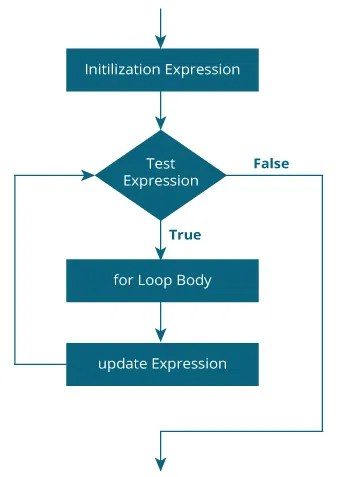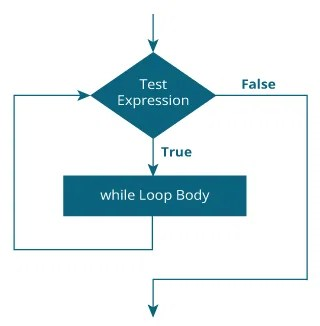# Fifth Chapter Lesson-16: Loop Control Statements in C programming language

## At the end of this lesson-

• 1. You will be able to explain loop.
• 2. You will be able to explain loop control statements.
• 3. You will be able to explain for, while and do-while loop control statements.
• 4. You will be able to differentiate between while and do-while loop control statements.

Loop: Loops are used in programming to repeat a block of code multiple times or until a specific condition is false.

Types of loop:

• 1. Infinite loop: The loop that repeat continuously and never end. A loop becomes an infinite loop if a condition never becomes false.
• 2. Finite loop : The loop that repeat for a particular times and it continues until a specific condition is false.
• 3. Nested loop: When one loop is inside another loop is called nested loop.

Loop Control Statements: A loop statement allows us to execute a statement or group of statements multiple times.

Types of loop control statement:

• 1. for loop statement
• 2. while loop statement
• 3. do…while loop statement

Each loop control statement is divided into two parts:

• Loop Declaration: There are three main parts of this section. Such as –
• Initialization Statement- In this expression we have to initialize the loop counter to some value. for example: int i=1;
• Test Expression- In this expression we have to test the condition. If the condition evaluates to true then we will execute the body of loop and go to update expression otherwise we will exit from the for loop. For example: i <= 10;
• Update Statement- After executing loop body this expression increments/decrements the loop variable by some value. for example: i++;
• Loop Body: The sequence of statements to be executed is kept inside the curly braces `{ }` known as the Loop body. it is is executed with each loop cycle.

Loop control statements are two types based on the position of loop_body and test_expression. They are-

1. Entry Controlled loops: In this type of loops the test condition is tested before entering the loop body. For Loop and While Loop are entry controlled loops.

2. Exit Controlled Loops: In this type of loops the test condition is tested or evaluated at the end of loop body. Therefore, the loop body will execute at least once, irrespective of whether the test condition is true or false. do – while loop is exit controlled loop.

for Loop Statement: `for` loop is used to execute a set of statements repeatedly until a particular condition is false. It tests the condition before executing the loop body. The for loop is commonly used when the number of iterations is known. Syntax of for loop-

### How for loop works-

1. The initialization statement is executed only once.

2. Then, the test expression is evaluated. If the test expression is true, codes inside the body of `for` loop is executed and the update expression is updated. This step-2 repeats until the test expression is false.

3. If the test expression is false, for loop is terminated.

Flowchart of for loop:Let’s see a program using for loop to print Hello World five times.

while loop statement: Executes a sequence of statements multiple times and abbreviates the code that manages the loop variable. It tests the condition before executing the loop body. while loops are used in situations where we do not know the exact number of iterations of loop beforehand. The loop execution is terminated on the basis of test condition. Syntax of while loop-

### How while loop works-

1. The while loop evaluates the test expression. If the test expression is true, codes inside the body of while loop is executed. The test expression is evaluated again. The process goes on until the test expression is false.

2. When the test expression is false, the while loop is terminated.

Flowchart of while loop:Syntax of while loop like for loop:

Let’s see a program using while loop to print Hello World five times.

do-while loop statement: The do-while loop is similar to the while loop with one important difference. The body of do-while loop is executed once, before checking the test expression. Hence, the do-while loop is executed at least once. Syntax of while loop-

### How do-while loop works-

1. The code block of loop body is executed once.

2. Then, the test expression is evaluated. If the test expression is true, the loop body is executed again. This process goes on until the test expression is false.

3. When the test expression is false, the do-while loop is terminated.

Flowchart of do-while loop:Syntax of do-while loop like for loop:

Let’s see a program using do-while loop to print Hello World five times.

## Lesson Evaluation-

Knowledge Based Questions:

• a. What is loop?
• a. What is loop control statement?
• a. What is entry control loop statement?
• a. What is exit control loop statement?

Comprehension Based Questions:

• b. Which one of for loop and while loop is more convenient to use?
• b. Explain the difference between while loop and do-while loop.

Creative Questions:

Multiple Choice Questions:

Written by,